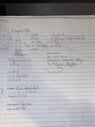# Conservation of Momentum of Rocket Exploding after Takeoff

• hisiks

#### hisiks

Homework Statement
A 1500 kg weather rocket accelerates upward at 10.0 m/s^2. It explodes 2.00 s after liftoff and
breaks into fragments. One piece is twice as massive as the other. Photos reveal that the
lighter fragment travelled straight up and reached a maximum height of 530 m. What was the
speed and direction of the heavier fragment just after the explosion?
Relevant Equations
pi = pf
m1v1i + m2v1i = m1v2f + m2v2f
vf = vi +at
d = vit + 1/2at^2
PEi + KEi = PEf + KEf
-Solved for vf using equation 3 to get 20.0m/s (speed before explosion) then solved for the distance to reach the explosion using equation 4, to get 20.0m, which felt wrong having the same numbers but that may just be coincidence.
-Found the distance travelled of the lighter piece using 530m - 20.0m to get 510m travelled after the explosion.
-Stuck trying to use equation 6 to find inital velocity.
Stuck with the remainder of the question.

EDIT: Found masses using Mtotal = m1 + m2, & m2 = 2m1, thefore m1 = 500kg and m2 = 1000kg.

Last edited:
that may just be coincidence
It's because the time is 2 seconds and ##\frac 122^2=2##.
Found masses
The actual masses are irrelevant, unless another part of the question asked for them. The given ratio is all you need.
What did you get for the speed and direction of the heavier portion?

It's because the time is 2 seconds and ##\frac 122^2=2##.

The actual masses are irrelevant, unless another part of the question asked for them. The given ratio is all you need.
What did you get for the speed and direction of the heavier portion?
This is what I came up with for a speed, however I'm not particularly confident in it. As for the direction, would it just be down?

#### Attachments

•image.jpg
37.8 KB · Views: 43
This is what I came up with for a speed, however I'm not particularly confident in it. As for the direction, would it just be down?
Looks right.

Looks right.
Would you agree the direction would just be down as well?

Would you agree the direction would just be down as well?
yes